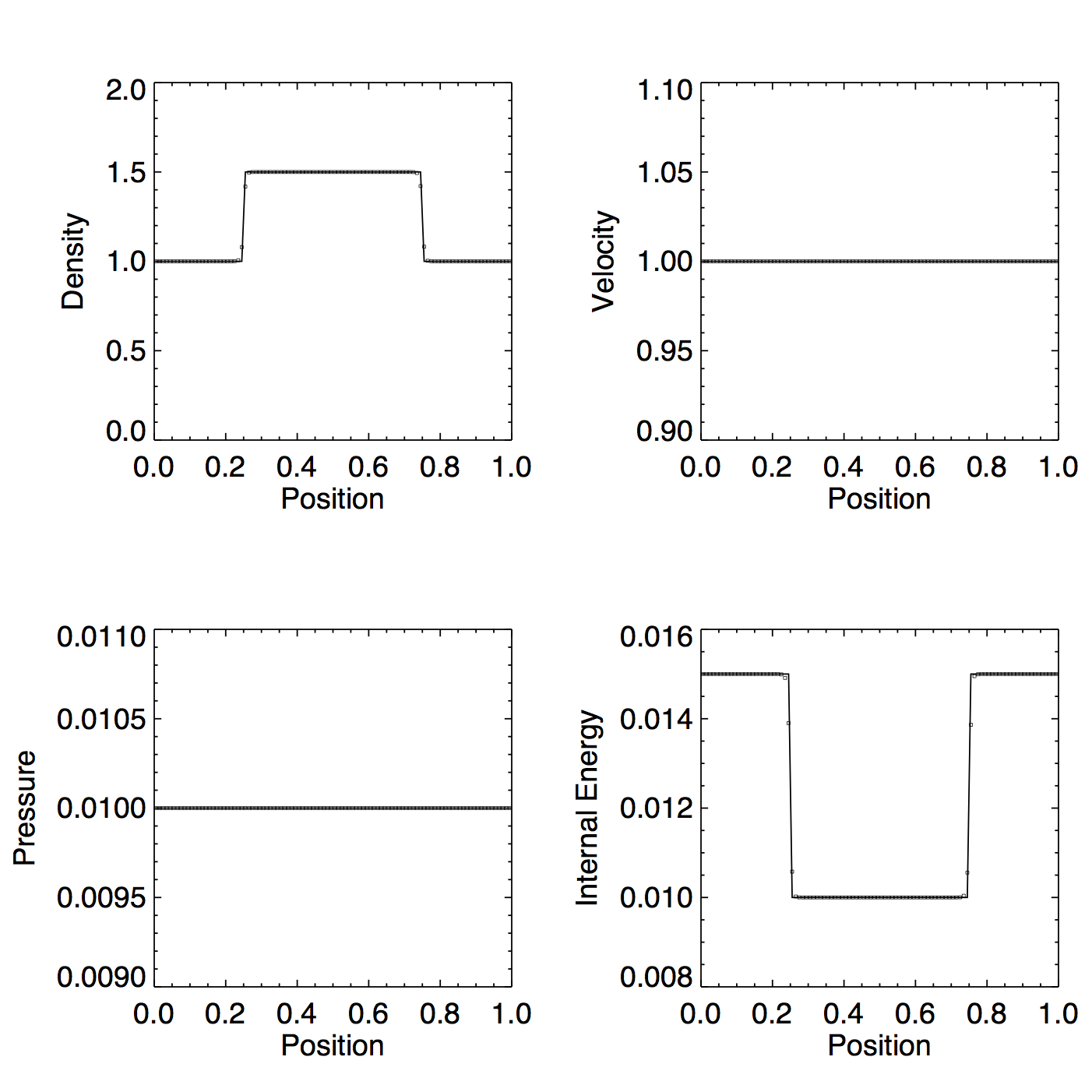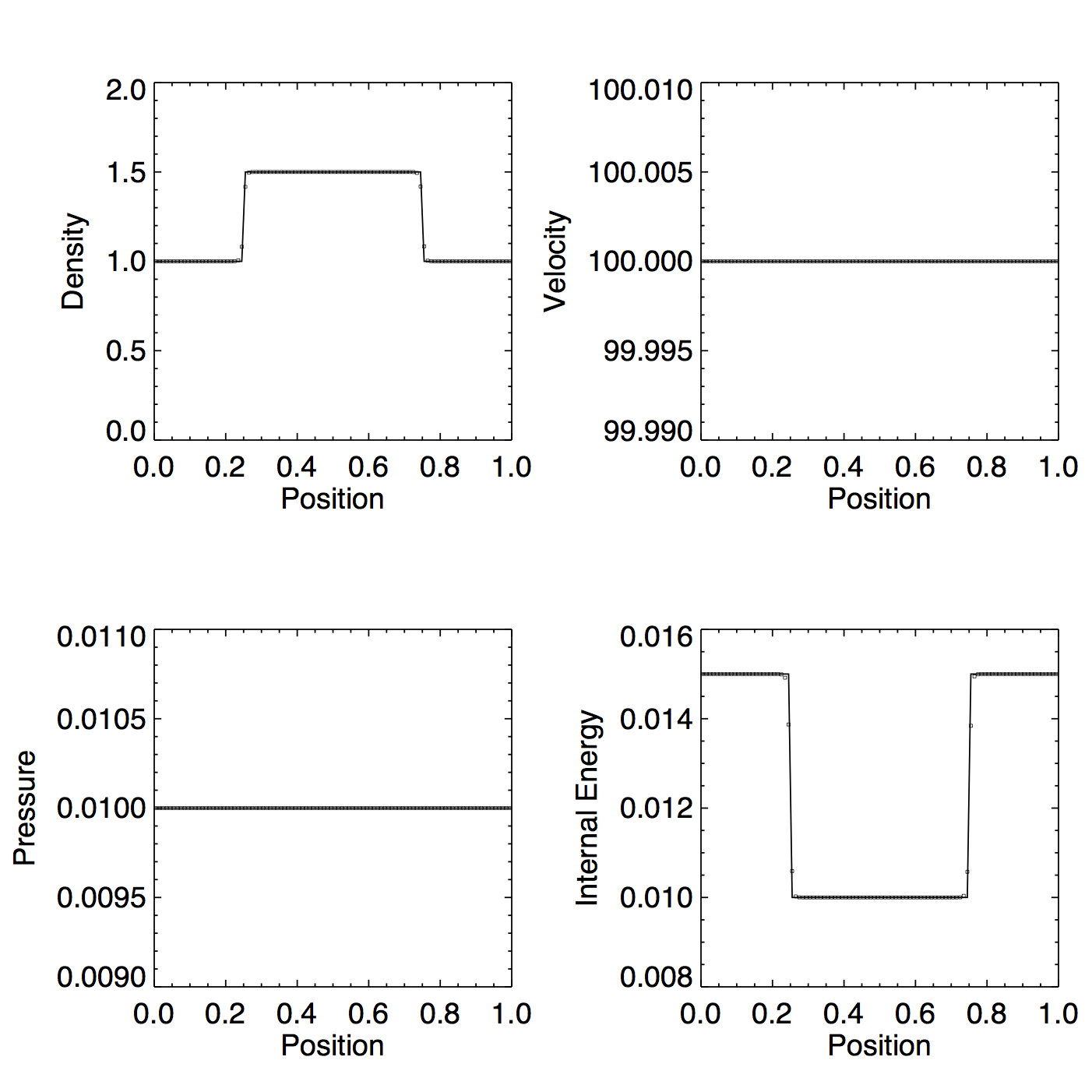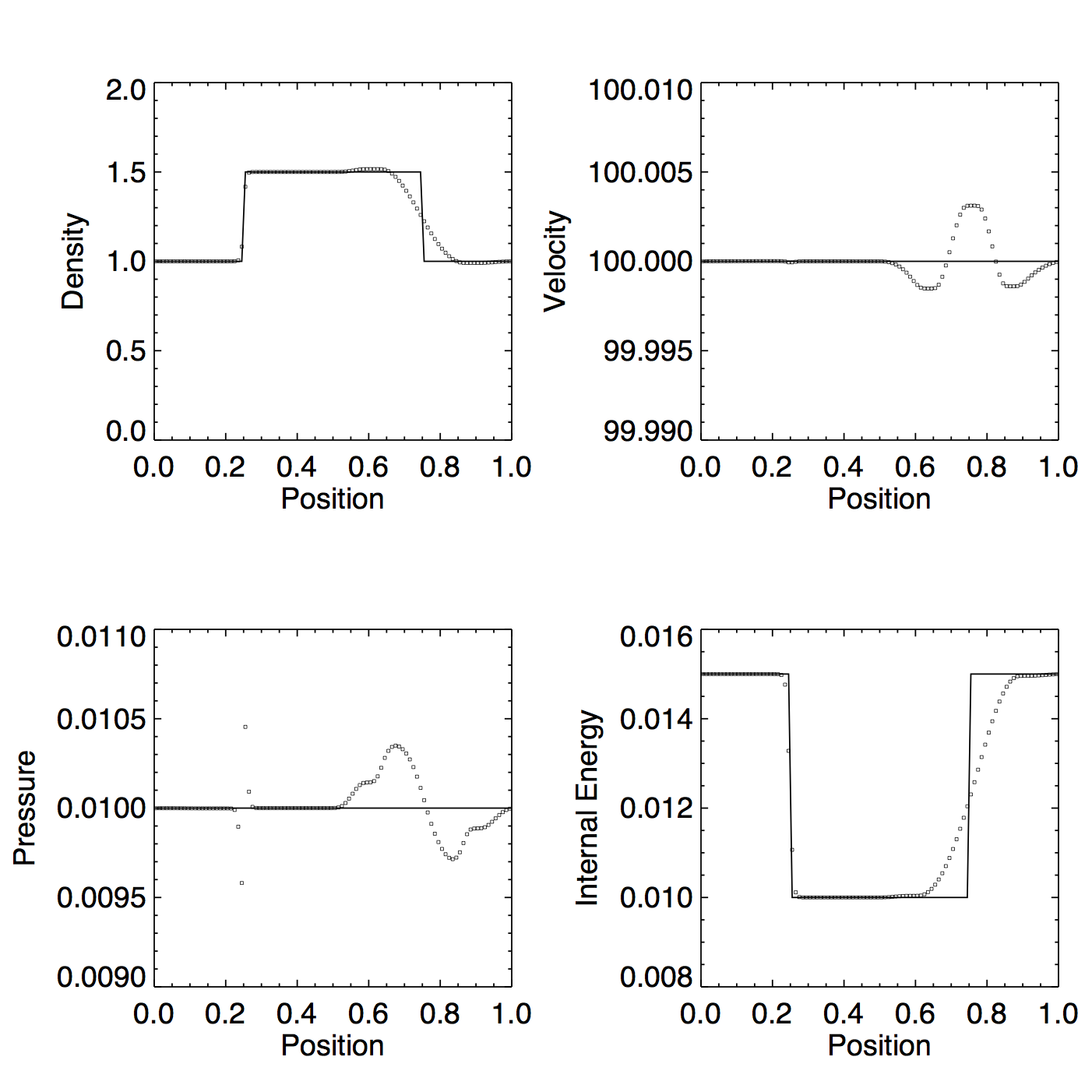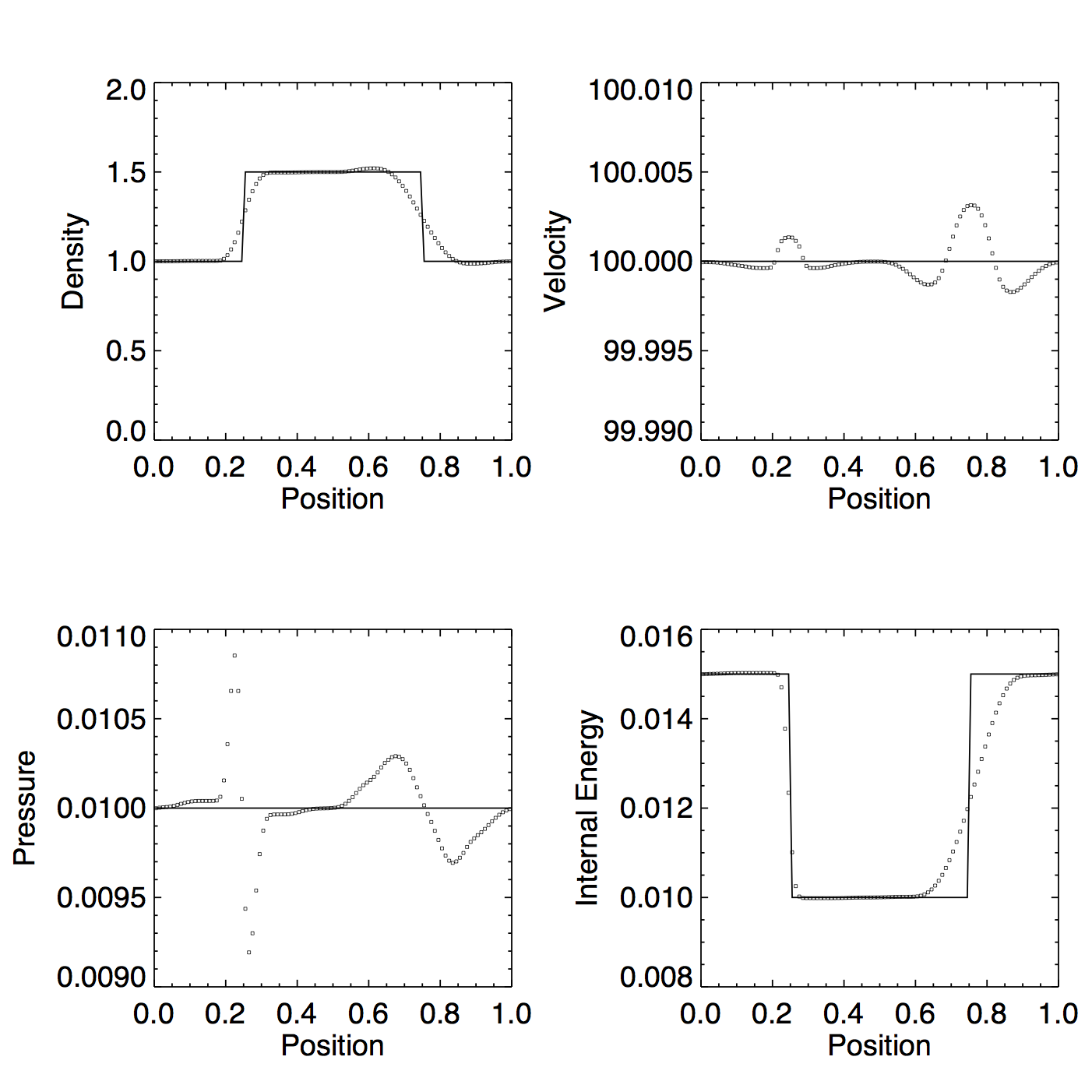# Dual Energy Tests (Part 3)

## October 1, 2015

In an effort to discover why the dual energy formalism is producing such unsatisfactory results on the high mach number shock tube, now I will look at a simpler test. In this post, I test an advected square wave (a density enhancement in pressure equilibrium moving across a periodic grid).

The tests

In the following tests, the pressure is $p = 0.01$ everywhere, $\gamma = \frac{5}{3}$, and density is $\rho = 1.0 * A$, where $A = 1.5$ in the middle section of the grid, and $A = 1.0$ elsewhere. $c_s = \sqrt{\frac{\gamma * p}{\rho}}$ ranges from 0.105 in the high density regions to 0.129 in the high density regions.

The domain goes from $x = [0, 1]$. For the low mach number test, $v = 1.0$, so the mach number is $M \approx 8$. For the high mach number test, $v = 100$, so the mach number is $M \approx 800$. The tests are run until $t = 1.0$, such that the low velocity test crosses the grid once, and the high velocity test crosses the grid 100 times.

Without Dual Energy

Figure 1: PPMP, exact solver, no dual energy, $v \approx 8 c_s$Figure 2: Same as Figure 1 but with $v \approx 800 c_s$PPMP fares quite well on this test, even at high mach number (not surprising, given that’s what it was designed to do). But what happens if we turn on the Enzo dual energy switch?

With Dual Energy

At low mach number, the switch doesn’t activate dual energy, and the results are identical to Figure 1. At high mach number $(v = 100 \approx 800 c_s)$, the switch kicks in, and the results are a mess.

Figure 3: Same as Figure 2 but with dual energyFigure 4: Same as Figure 4 but with PPMCThe Internal Energy Update

The equation that is used to update the internal energy has two parts: a term that represents the advected flux of internal energy, and a term that represents the gain or loss of internal energy due to pressure and velocity gradients. In this case, the velocity is equal across all cells, so the second term should be 0. For the first time step, this criterion holds, but by the second, there are already a few cells which show a very small velocity gradient.

Is this because the first term isn’t acting properly? Or because it’s simply not accurate enough?